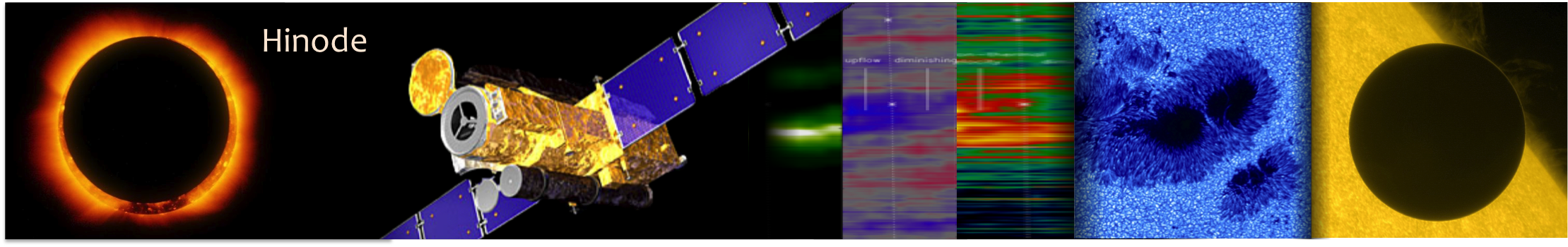# National Aeronautics and Space Administration

## Marshall Space Flight Center# Hinode (Solar-B)

## Interactive Resources

1. Astrophysics Calculator
- A neat calculator at the University of Wisconsin with many built-in astronomical constants. [Java]

2. USNO Sun and Moon Calculator
- Enter your location on Earth and it calculates sunrise, sunset etc.

3. Distance between points on Earth
- Has nothing to do with the sun, but it is pretty fun to use!

4. Cosmology Calculator
- Has nothing to do with the sun, but again it is very useful to us astronomers.

5. Sample Size Calculator
- Tells you how big your sample needs to be to get to a certain level of statistical confidence. Great resource to apply to data and meaasurements to make sure your answers are statistically significant.

6. Radiation Dose and Risk Calculator
- Converts exposure and dosage in Seiverts, Greys, Roentgens etc, into risks for cancer.

7. Ohms Law Calculator
- Convert currents, voltages, resistences solving E = I x R.

8. Earth's Magnetic Field Calculator
- Find the strength of Earth's field anywhere from on the surface into space.

9. Frequency and Wavelength Calculator
- For electromagnetic radiation. It also gives the energy of each photon.

10. Mass to Energy Calculator
- Converts mass to energy using Einstein's Famous Formula.

11. Electron Voltage to Electron Properties Calculator
- If you imnput the energy of the electron in Volts, the calculator tells you it speed, mass, momentum and DeBroglie wavelength.

12. Units Converter
- For energy, speed, length, mass etc.

13. Magnetic Unit Converter
- Converts from one unit into many others.

14. Energy and Power Conversion
- Converts Joules and kilowatts into other units.